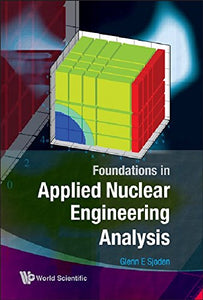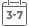# Foundations In Applied Nuclear Engineering Analysis

• Publish Date: 2009-07-15
• Binding: Paperback
• Author: Glenn E. Sjoden
Regular price \$60.29 Sale price \$38.82

Attention: For textbook, access codes and supplements are not guaranteed with used items.This text addresses a number of technical skills in mathematics, physics, and specific areas of nuclear engineering that will prepare the student for optimum performance in any nuclear engineering or medical physics curriculum. The book opens with fundamentals in probability and statistics, ODEs, series solutions, general differential equations, numerical methods, up through PDEs, and incorporates modeling and simulation, radiation, heat transfer, neutron diffusion problems, advanced solution methods, and engineering problem solving. The book specifically focuses on examples in nuclear and radiological engineering, and is thus a unique text for nuclear engineering students. A course using the book may range from three to four credits. Several applications in Mathematica are written to illustrate technical concepts.

• Nuclear Engineering Analysis
• Essentials of Probability
• Introduction to Numerical Concepts and Applications
• Complex Numbers Fundamentals
• Methods for Solving Ordinary Differential Equations
• Applications of Power Series
• Solution Methods for Differential Equations with Variable Coefficients
• Vectors, Matrices, and Linear Systems
• Gram-Schmidt Orthogonalization and Fourier Series
• Applied Solution Methods -- Part 1
• Applied Solution Methods -- Part 2
• Numerical Solutions of Partial Differential Equations
• Appendix: Selected Problems in Applied Nuclear Engineering Analysis